# 235 Results

View
Selected filters:
• Calculus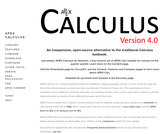Conditional Remix & Share Permitted
CC BY-NC
Rating

APEX Calculus is a calculus textbook written for traditional college/university calculus courses. It has the look and feel of the calculus book you likely use right now (Stewart, Thomas & Finney, etc.). The explanations of new concepts is clear, written for someone who does not yet know calculus. Each section ends with an exercise set with ample problems to practice & test skills (odd answers are in the back).

Subject:
Calculus
Material Type:
Textbook
Provider:
APEX: Affordable Print and Electronic TeXtbooks
Author:
Brian Heinold
Dimplekumar Chalishajar
Gregory Hartman
Troy Siemers
01/01/2014Conditional Remix & Share Permitted
CC BY-NC-SA
Rating

Active Calculus is different from most existing calculus texts in at least the following ways: the text is free for download by students and instructors in .pdf format; in the electronic format, graphics are in full color and there are live html links to java applets; the text is open source, and interested instructors can gain access to the original source files upon request; the style of the text requires students to be active learners — there are very few worked examples in the text, with there instead being 3-4 activities per section that engage students in connecting ideas, solving problems, and developing understanding of key calculus concepts; each section begins with motivating questions, a brief introduction, and a preview activity, all of which are designed to be read and completed prior to class; the exercises are few in number and challenging in nature.

Subject:
Mathematics
Calculus
Material Type:
Textbook
Provider:
Grand Valley State University
Author:
David Austin
Matt Boelkins
Steve Schlicker
05/22/2019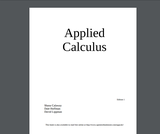Unrestricted Use
CC BY
Rating

An openly licensed applied calculus textbook, covering derivatives, integrals, and an intro to multivariable calculus. This book is heavily remixed from Dale Hoffman's Contemporary Calculus textbook, and retains the same conceptual focus from that text.

Subject:
Mathematics
Calculus
Material Type:
Textbook
Author:
Dale Hoffman
David Lippman
Shana Calaway
10/22/2019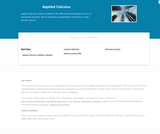Rating

Applied Calculus instructs students in the differential and integral calculus of elementary functions with an emphasis on applications to business, social and life science. Different from a traditional calculus course for engineering, science and math majors, this course does not use trigonometry, nor does it focus on mathematical proofs as an instructional method.

Subject:
Calculus
Material Type:
Full Course
Textbook
Provider:
Lumen Learning
Provider Set:
Candela Courseware
Author:
Dale Hoffman
David Lippman
Shana Calaway
05/22/2019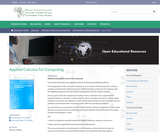Unrestricted Use
CC BY
Rating

Welcome to applied calculus for computing. Calculation intended to be a general method of solving quantifiable problems. In the application of the calculation method, or as it is known the”infinitesimal” method, a problem is”divided into infinitesimal parts”(differentiation), analysed in its relations with the neighbouring parts and then”added”(integration) until the solution method. The two parts of this the analysis and synthesis form a model for more sophisticated methods based on calculation, used in applied science concepts you learn in calculus allow statistical , physicists and engineers create mathematical models of real situations and real problems and simulate their resolutions under different operating conditions.

Subject:
Calculus
Material Type:
Module
Provider:
African Virtual University
Provider Set:
OER@AVU
Author:
Willian Munyiri
02/10/2018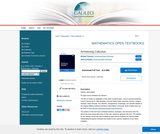Conditional Remix & Share Permitted
CC BY-NC-SA
Rating

The text is mostly an adaptation of two other excellent open- source calculus textbooks: Active Calculus by Dr. Matt Boelkins of Grand Valley State University and Drs. Gregory Hartman, Brian Heinold, Troy Siemers, Dimplekumar Chalishajar, and Jennifer Bowen of the Virginia Military Institute and Mount Saint Mary's University. Both of these texts can be found at http://aimath.org/textbooks/approved-textbooks/.
The authors of this text have combined sections, examples, and exercises from the above two texts along with some of their own content to generate this text. The impetus for the creation of this text was to adopt an open-source textbook for Calculus while maintaining the typical schedule and content of the calculus sequence at our home institution.

Subject:
Calculus
Material Type:
Textbook
Provider:
University System of Georgia
Provider Set:
Galileo Open Learning Materials
Author:
Jared Schlieper,
Michael Tiemeyer
03/20/2015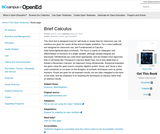Unrestricted Use
CC BY
Rating

This short text is designed more for self-study or review than for classroom use; full solutions are given for nearly all the end-of-chapter problems. For a more traditional text designed for classroom use, see Fundamentals of Calculus (http://www.lightandmatter.com/fund/). The focus is mainly on integration and differentiation of functions of a single variable, although iterated integrals are discussed. Infinitesimals are used when appropriate, and are treated more rigorously than in old books like Thompson's Calculus Made Easy, but in less detail than in Keisler's Elementary Calculus: An Approach Using Infinitesimals. Numerical examples are given using the open-source computer algebra system Yacas, and Yacas is also used sometimes to cut down on the drudgery of symbolic techniques such as partial fractions. Proofs are given for all important results, but are often relegated to the back of the book, and the emphasis is on teaching the techniques of calculus rather than on abstract results.

Subject:
Mathematics
Calculus
Material Type:
Textbook
Provider:
BCcampus
Provider Set:
BCcampus Open Textbooks
Author:
Benjamin Crowell
10/28/2014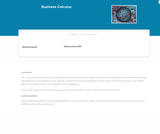Rating

This course provides an introduction to applied concepts in Calculus that are relevant to the managerial, life, and social sciences. Students should have a firm grasp of the concept of functions to succeed in this course. Topics covered include derivatives of basic functions and how they can be used to optimize quantities such as profit and revenues, as well as integrals of basic functions and how they can be used to describe the total change in a quantity over time.

Subject:
Calculus
Material Type:
Full Course
Textbook
Provider:
Lumen Learning
Provider Set:
Candela Courseware
05/22/2019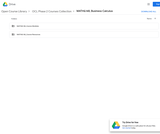Unrestricted Use
CC BY
Rating

MATH&148 is a calculus course for business students. It is designed for students who want a brief course in calculus. Topics include differential and integral calculus of elementary functions. Problems emphasize business and social science applications. Translating words into mathematics and solving word problems are emphasized over algebra. Applications are mainly business oriented (e.g. cost, revenue, and profit). Mathematical theory and complex algebraic manipulations are not mainstays of this course, which is designed to be less rigorous than the calculus sequence for scientists and engineers. Topics are presented according to the rule of four: geometrically, numerically, analytically, and verbally. That is, symbolic manipulation must be balanced with graphical interpretation, numerical examples, and writing. Trigonometry is not part of the course.

Subject:
Calculus
Material Type:
Assessment
Full Course
Syllabus
Textbook
Provider:
Washington State Board for Community & Technical Colleges
Provider Set:
Open Course Library
05/22/2019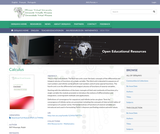Unrestricted Use
CC BY
Rating

This is a four unit module. The first two units cover the basic concepts of the differential and integral calculus of functions of a single variable. The third unit is devoted to sequences of real numbers and infinite series of both real numbers and of some special functions. The fourth unit is on the differential and integral calculus of functions of several variables.

Subject:
Calculus
Material Type:
Module
Provider:
African Virtual University
Provider Set:
OER@AVU
Author:
Ralph W.P. Masenge
03/14/2018Conditional Remix & Share Permitted
CC BY-NC-SA
Rating

Published in 1991 by Wellesley-Cambridge Press, the book is a useful resource for educators and self-learners alike. It is well organized, covers single variable and multivariable calculus in depth, and is rich with applications.

In addition to the Textbook, there is also an online Instructor's Manual and a student Study Guide. Prof. Strang has also developed a related series of videos, Highlights of Calculus, on the basic ideas of calculus.

Subject:
Mathematics
Calculus
Material Type:
Full Course
Provider:
M.I.T.
Author:
Gilbert Strang
01/01/1991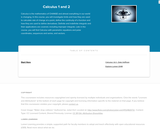Rating

Calculus is the mathematics of CHANGE and almost everything in our world is changing. In this course, you will investigate limits and how they are used to calculate rate of change at a point, define the continuity of a function and how they are used to define derivatives. Definite and indefinite integrals and their applications are covered, including improper integrals. Late in the course, you will find Calculus with parametric equations and polar coordinates, sequences and series, and vectors.

Subject:
Calculus
Material Type:
Full Course
Textbook
Provider:
Lumen Learning
Provider Set:
Candela Courseware
Author:
Dale Hoffman
David Lippman
Jeff Eldridge
Melonie Rasmussen
05/22/2019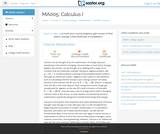Unrestricted Use
CC BY
Rating

This course begins with a review of algebra specifically designed to help and prepare the student for the study of calculus, and continues with discussion of functions, graphs, limits, continuity, and derivatives. The appendix provides a large collection of reference facts, geometry, and trigonometry that will assist in solving calculus problems long after the course is over. Upon successful completion of this course, the student will be able to: calculate or estimate limits of functions given by formulas, graphs, or tables by using properties of limits and LĺÎĺ_ĺĚĺ_hopitalĺÎĺ_ĺĚĺ_s Rule; state whether a function given by a graph or formula is continuous or differentiable at a given point or on a given interval and justify the answer; calculate average and instantaneous rates of change in context, and state the meaning and units of the derivative for functions given graphically; calculate derivatives of polynomial, rational, common transcendental functions, and implicitly defined functions; apply the ideas and techniques of derivatives to solve maximum and minimum problems and related rate problems, and calculate slopes and rates for function given as parametric equations; find extreme values of modeling functions given by formulas or graphs; predict, construct, and interpret the shapes of graphs; solve equations using NewtonĺÎĺ_ĺĚĺ_s Method; find linear approximations to functions using differentials; festate in words the meanings of the solutions to applied problems, attaching the appropriate units to an answer; state which parts of a mathematical statement are assumptions, such as hypotheses, and which parts are conclusions. This free course may be completed online at any time. It has been developed through a partnership with the Washington State Board for Community and Technical Colleges; the Saylor Foundation has modified some WSBCTC materials. (Mathematics 005)

Subject:
Calculus
Functions
Material Type:
Assessment
Full Course
Syllabus
Textbook
Provider:
The Saylor Foundation
10/24/2019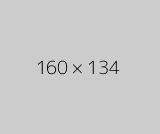Conditional Remix & Share Permitted
CC BY-NC
Rating

The Calculus I course was developed through the Ohio Department of Higher Education OER Innovation Grant. This work was completed and the course was posted in February 2019. The course is part of the Ohio Transfer Module and is also named TMM005. For more information about credit transfer between Ohio colleges and universities, please visit: www.ohiohighered.org/transfer.Team LeadJim Fowler                                         Ohio State UniversityRita Ralph                                         Columbus State Community CollegeContent ContributorsNela Lakos                                       Ohio State UniversityBart Snapp                                       Ohio State UniversityJames Talamo                                  Ohio State UniversityXiang Yan                                         Edison State Community CollegeLibrarianDaniel Dotson                                    Ohio State University                     Review TeamThomas Needham                             Ohio State UniversityCarl Stitz                                            Lakeland Community CollegeSara Rollo                                          North Central State College

Subject:
Mathematics
Calculus
Material Type:
Full Course
Provider:
Ohio Open Ed Collaborative
05/07/2021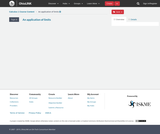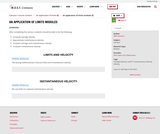Conditional Remix & Share Permitted
CC BY-NC
Rating

After completing this section, students should be able to do the following.Compute average velocity.Approximate instantaneous velocity.Compare average and instantaneous velocity.Compute instantaneous velocity.

Subject:
Calculus
Material Type:
Module
Author:
OER Librarian
05/07/2021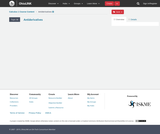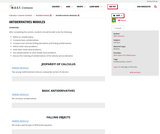Conditional Remix & Share Permitted
CC BY-NC
Rating

After completing this section, students should be able to do the following.Define an antiderivative.Compute basic antiderivatives.Compare and contrast finding derivatives and finding antiderivatives.Define initial value problems.Solve basic initial value problems.Use antiderivatives to solve simple word problems.Discuss the meaning of antiderivatives of the velocity and acceleration.

Subject:
Calculus
Material Type:
Module
Author:
OER Librarian
05/07/2021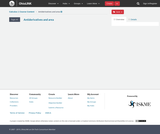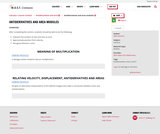Conditional Remix & Share Permitted
CC BY-NC
Rating

After completing this section, students should be able to do the following.Interpert the product of rate and time as area.Approximate position from velocity.Recognize Riemann sums.

Subject:
Calculus
Material Type:
Module
Author:
OER Librarian